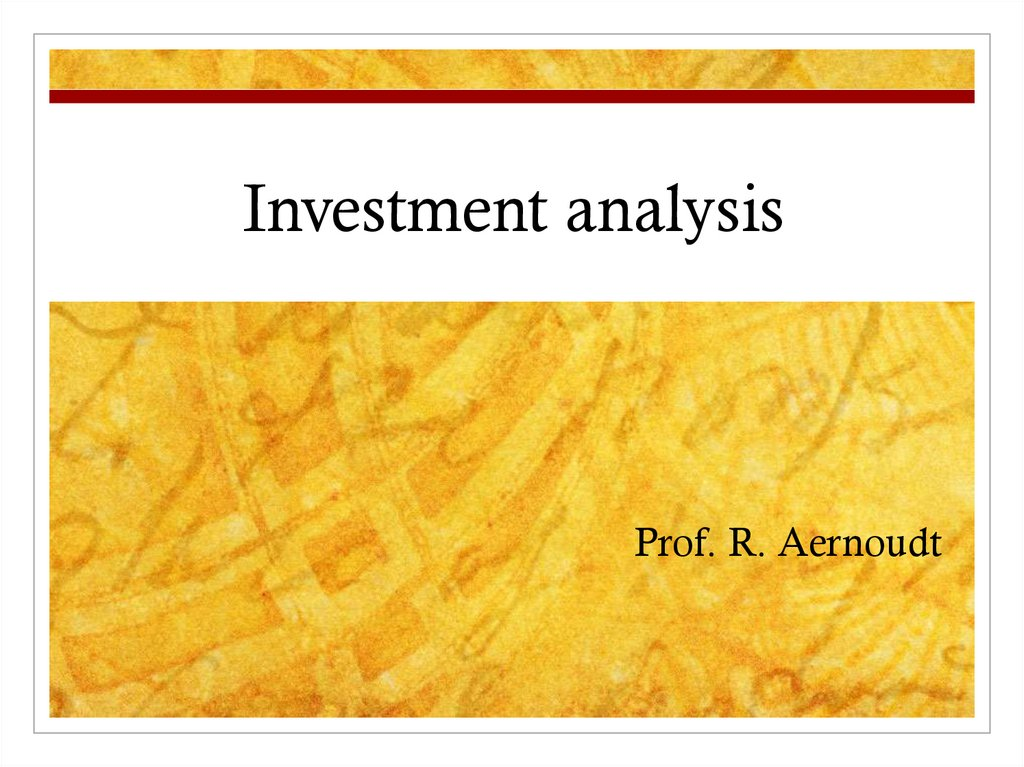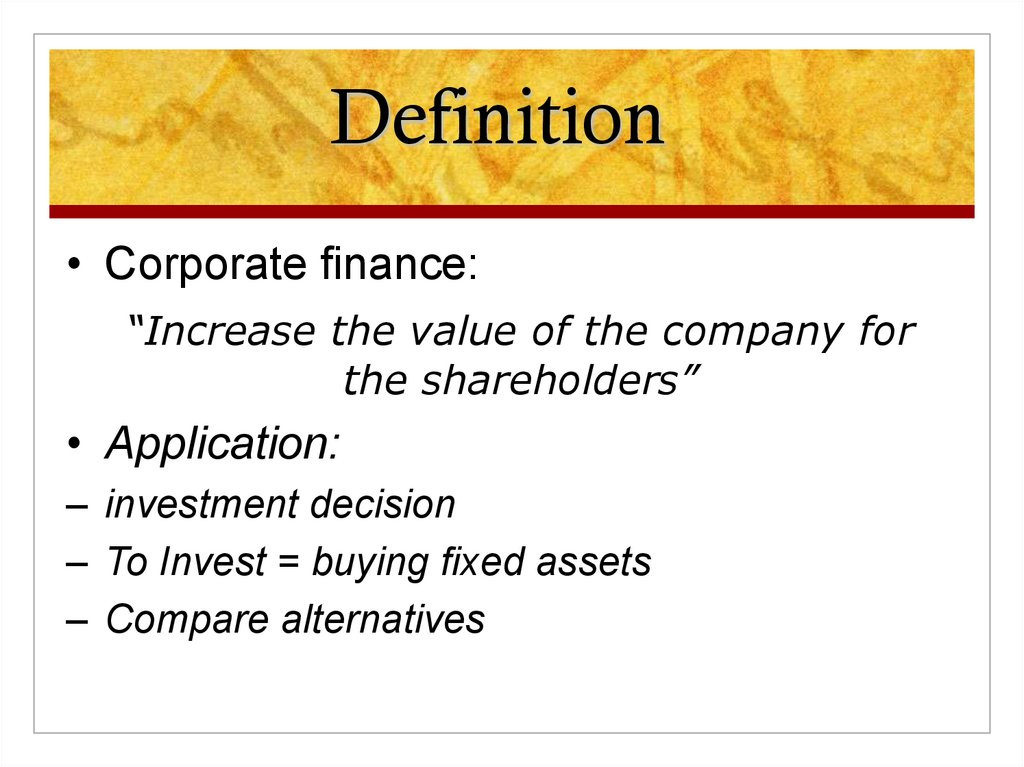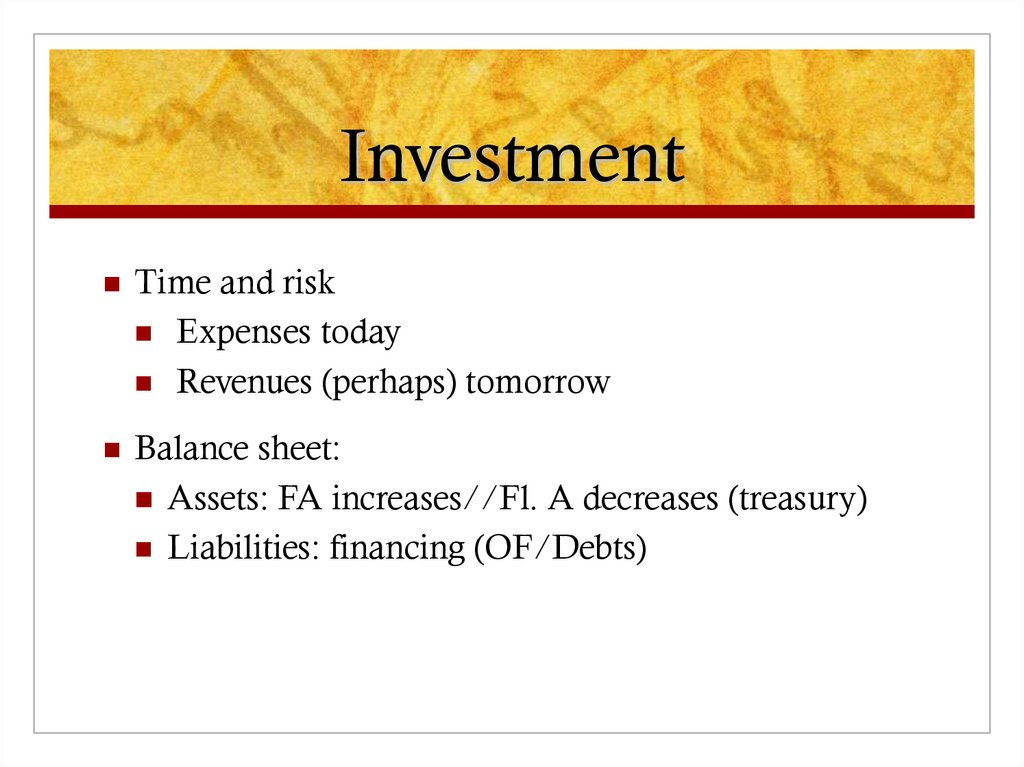# Investment analysis

## 1. Investment analysis

Prof. R. Aernoudt

## 2. Definition

• Corporate finance:
“Increase the value of the company for
the shareholders”
• Application:
– investment decision
– To Invest = buying fixed assets
– Compare alternatives

## 3. Investment

Time and risk
Expenses today
Revenues (perhaps) tomorrow
Balance sheet:
Assets: FA increases//Fl. A decreases (treasury)
Liabilities: financing (OF/Debts)

## 4. Investment Hermès Ltd

Actual value of investment:
Yearly generated CF: 5720
Should we do the investment?
Different methods:
Payback period
IRR
Net actual value
Profitability index (PI)
12700

## 5. 1/ Payback period

TVP = Initial investment/CF
12 700/5720 = 2,2 year
Inconvenients:
What happens afterwards ?
Length = arbitrary
CFs are not actualised
Easy method
Used quite a lot

## 6. Internal rate of return

IRR
Return where actuak value of expected CIFs equals the
present value of expected COFs.
BI = CF1 + CF2 + CF3 + …. = ΣCFn
1+r
Annuity
(1 + r)²
(1+r)³
(1 + r)ⁿ

## 7. Internal rate of return (2)

Annuity = what is the actual value of an amount that I
get every year?
CF 5720//Inv. 12070
12070 = ann. factor x 5720
See annuity tables
2,1427
Cutoff rate of hurdle rate
37%, 2,1058
38%

## 8. Internal Rate of Return (3)

Inconvenient of method:
Difficult to calculate
How to fix cut-off rate
Easy to compare projects
Actualisation of returns

## 9. 3. Net actual value

Ex ante fixed minimum return (v)
COF ≥ CIF: not invest
NAV = ΣCIF/(1 + v)ⁿ - ΣCOF/(1 + v)ⁿ
Suppose 40%: 5720 x ann. Factor (2,0352)
CIF – COF: 11641 – 12070 = negative
Inconvenient:
As complicated as IRR
Difficult to compare alternatives
How to fix v?

## 10. 4. Profitability index

Variation on same topic
PI = ΣCIF/(1 + v)ⁿ
ΣCOF/(1 + v)ⁿ
If PI ≥ 1 then invest
Inconvenient: idem NAV
Exercise: calculate PI with required return of 35%.

## 11. Case : Ltd Hermès

Turnover: year n +1: 200 M; thereafter 250 M
Financing proposition bank:
1st phase: 3700 K; 5 years
2nd phase: 8000 K; 8 years (not 10)
Evaluate investment following different methods
Make exercises 5 & 6